# Groups

In the 6th class there are 60 girls and 72 boys. We want to divide them into groups so that the number of girls and boys is the same. How many groups can you create? How many girls will be in the group?

n =  11
d =  5

### Step-by-step explanation:

$d=d\mathrm{/}a=5\mathrm{/}12=5$Did you find an error or inaccuracy? Feel free to write us. Thank you!Tips to related online calculators
Do you want to calculate greatest common divisor two or more numbers?
Do you want to perform natural numbers division - find the quotient and remainder?

## Related math problems and questions:

• Sports studentsThere are 120 athletes, 48 volleyball players, and 72 handball players at the school with extended sports training. Is it possible to divide sports students into groups so that the number in each group is the same and expressed by the largest possible num
• Two hundred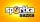Two hundred ten athletes competed in three athletics races on three fields. One hundred five athletes competed in the first, 60 in the second, and everyone else in the third. On the individual courts, the athletes were divided into groups. Each group, alt
• SegmentsLine segments 62 cm and 2.2 dm long we divide into equal parts which lengths in centimeters is expressed integer. How many ways can we divide?
• Athletics teamAll athletes in the athletics team from Ostrava can start in four, five, six and seven stages, and no one will be present. How many average athletes are in one athletic group if there are a total of twelve groups in the section? Consider the smallest numb
• TilesHall has dimensions 325 &time; 170 dm. What is the largest size of square tiles that can be entire hall tiled, and how many we need them?
• Trees in alleyThere are four trees in the alley between which the distances are 35m, 15m and 95m. Trees must be laid in the spaces so that the distance is the same and the maximum. How many trees will they put in and what will be the distance between them?
• Gcd and lcm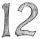Calculate the greatest common divisor and the least common multiple of numbers. a) 16 and 18 b) 24 and 22 c) 45 and 60 d) 36 and 30
• PlumsIn the bowl are plums. How many would be there if we can divide it equally among 8, 10 and 11 children?
• Part-time workers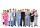On Saturday, 210 part-time workers arrived in the village. One hundred five part-time workers worked on the first field, 60 on the second. The other part-time workers worked in the third field. They all worked in equal groups. How many workers worked in e
• PegsFrom two sticks 240 cm and 210 cm long, it is necessary to cut the longest possible pegs for flowers so that no residues remain. How many pegs will it be?
• Rectangular flowerbedAround the rectangular flowerbed with dimensions of 5.25 m and 3.50 m, roses should be planted at the same distance from each other so that the roses are located in each corner of the flower bed and are consumed as little as possible. How far do we plant
• Florist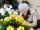Florist has 84 red and 48 white roses. How many same bouquets can he make from them if he must use all roses?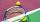The children of the tennis school received 64 white and 48 yellow balls from the sponsor. When asked about how many balls they could take, they answered: "You have so many that none of you will have more than ten balls, and all will have the same number o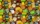We bought 140 fruit, 196 chocolate and 84 caramel lollipops. How many of the same packages can we prepare from them?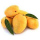Chris has 12 mangoes and Jay has 18 mangoes. Each of them will share the mangoes to their friends . What's the greatest number of mangoes each of their friends get if Chris and Jay will give the same number of mangoes?On Children's Day, the organizers bought 252 chewing gums, 396 candies and 108 lollipops. They want to make as many of the same packages as possible. Advise them what to put in each package and how many packages they can make this way.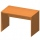In the two dining rooms in the recreational building, there are equally arranged chairs around the tables. A maximum of 78 people can dine in the first dining room and 54 people in the second. How many chairs can be around one table?